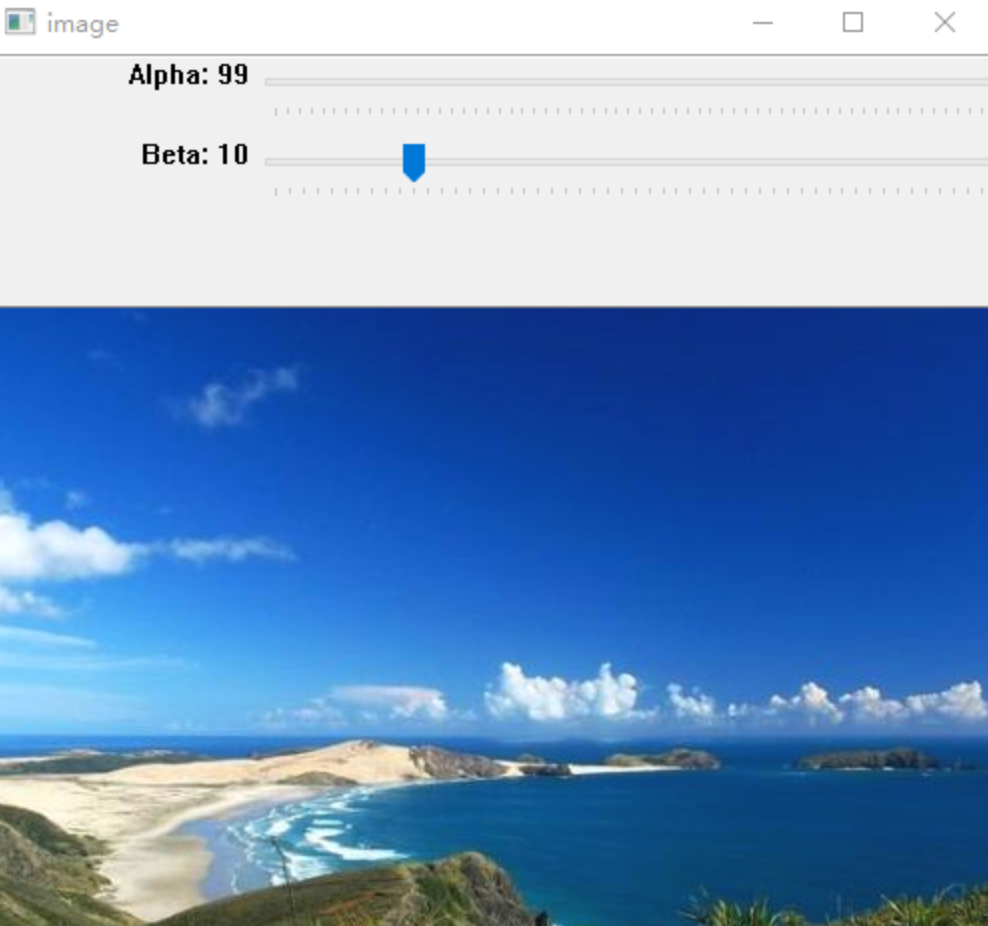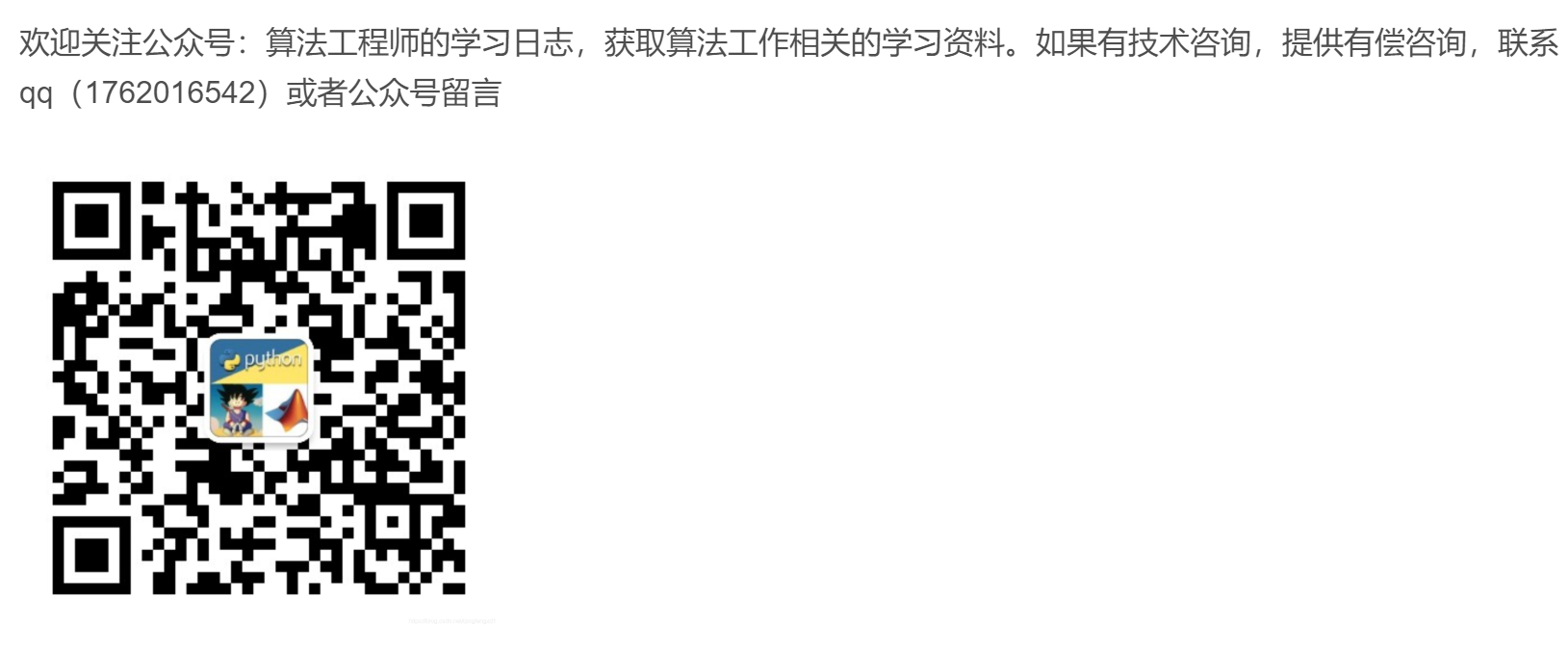import cv2
import numpy as np
# 图像混合
dst = cv2.addWeighted(img1, 0.7, img2, 0.3, 0)
# 第一幅图的权重是 0.7 第二幅图的权重是 0.3。大概就是第二幅图浮现加在第一个图上
#g(x) = αf(x) + β，其中：α(>0)、β常称为增益与偏置值，分别控制图片的对比度和亮度。
res = np.uint8(np.clip((1.5 * dst + 10), 0, 255)) # 1.5是对比度   10是亮度
cv2.imwrite("res.png",res) #保存
cv2.imshow('dst', res)    #显示
cv2.waitKey(0)
cv2.destroyAllWindows()


展开全文opencv
• 基本原理：两张图片合成。  先按照原来的图片的格式新建一个色素全为零的图片，然后按照两张图的比例不同合成一张新图片。...#粗略的调节对比度和亮度 def contrast_brightness_image(src1, a, g): ...
基本原理：两张图片合成。
# -*- coding=GBK -*-
import cv2 as cv
import numpy as np

#粗略的调节对比度和亮度
def contrast_brightness_image(src1, a, g):
h, w, ch = src1.shape#获取shape的数值，height和width、通道

#新建全零图片数组src2,将height和width，类型设置为原图片的通道类型(色素全为零，输出为全黑图片)
src2 = np.zeros([h, w, ch], src1.dtype)
cv.imshow("con-bri-demo", dst)

cv.namedWindow("原来", cv.WINDOW_NORMAL)
cv.imshow("原来", src)
contrast_brightness_image(src, 1.2, 10)#第一个1.2为对比度  第二个为亮度数值越大越亮
cv.waitKey(0)
cv.destroyAllWindows()
运行结果如下：addWeighted(InputArray src1, double alpha, InputArray src2, double beta, double gamma, OutputArray dst, int dtype=-1);
一共有七个参数：前4个是两张要合成的图片及它们所占比例，第5个double gamma起微调作用，第6个OutputArray dst是合成后的图片，第七个输出的图片的类型（可选参数，默认-1）
有公式得出两个图片加成输出的图片为：dst=src1*alpha+src2*beta+gamma
展开全文• python-OpenCV图像处理，图像对比度调节源代码。
• python opencv调节图片亮度与对比度调整是将图像像素的强度整体变大/变小，对比度调整指的是图像暗处变得更暗，亮出变得更亮，从而拓宽某个区域内的显示精度。创建两个滑动条分别调整对比度和亮度（对比度范围...
python opencv调节图片亮度与对比度

度调整是将图像像素的强度整体变大/变小，对比度调整指的是图像暗处变得更暗，亮出变得更亮，从而拓宽某个区域内的显示精度。创建两个滑动条分别调整对比度和亮度（对比度范围：0 ~ 0.3， 亮度0 ~ 100）。提示：因为滑动条没有小数，所以可以设置为0 ~ 300，然后乘以0.01
代码
import cv2
import numpy as np
alpha = 0.3
beta = 80
img_path = "7MeansDenoising/1_1.bmp"
def updateAlpha(x):
global alpha, img, img2
alpha = cv2.getTrackbarPos('Alpha', 'image')
alpha = alpha * 0.01
img = np.uint8(np.clip((alpha * img2 + beta), 0, 255))
def updateBeta(x):
global beta, img, img2
beta = cv2.getTrackbarPos('Beta', 'image')
img = np.uint8(np.clip((alpha * img2 + beta), 0, 255))
# 创建窗口
cv2.namedWindow('image')
cv2.createTrackbar('Alpha', 'image', 0, 300, updateAlpha)
cv2.createTrackbar('Beta', 'image', 0, 255, updateBeta)
cv2.setTrackbarPos('Alpha', 'image', 100)
cv2.setTrackbarPos('Beta', 'image', 10)
while (True):
cv2.imshow('image', img)
if cv2.waitKey(1) == ord('q'):
break
cv2.destroyAllWindows()

效果展开全文opencv 亮度
• 声明：本文转自...图像亮度与对比度调整，是放在skimage包的exposure模块里面 1、gamma调整 原理：I=Ig 对原图像的像素，进行幂运算，得到新的像素值。公式中的g就是gamma值。 如果g
声明：本文转自http://blog.csdn.NET/haoji007/article/category/6337049，但其也为转载，原作者不明，感谢原作者的付出和分享。

图像亮度与对比度的调整，是放在skimage包的exposure模块里面
1、gamma调整
原理：I=Ig
对原图像的像素，进行幂运算，得到新的像素值。公式中的g就是gamma值。
如果gamma>1, 新图像比原图像暗
如果gamma<1,新图像比原图像亮
gamma参数默认为1，原像不发生变化 。from skimage import data, exposure, img_as_float
import matplotlib.pyplot as plt
image = img_as_float(data.moon())

plt.subplot(131)
plt.title('origin image')
plt.imshow(image,plt.cm.gray)
plt.axis('off')

plt.subplot(132)
plt.title('gamma=2')
plt.imshow(gam1,plt.cm.gray)
plt.axis('off')

plt.subplot(133)
plt.title('gamma=0.5')
plt.imshow(gam2,plt.cm.gray)
plt.axis('off')

plt.show()2、log对数调整
这个刚好和gamma相反
原理：I=log(I)from skimage import data, exposure, img_as_float
import matplotlib.pyplot as plt
image = img_as_float(data.moon())

plt.subplot(121)
plt.title('origin image')
plt.imshow(image,plt.cm.gray)
plt.axis('off')

plt.subplot(122)
plt.title('log')
plt.imshow(gam1,plt.cm.gray)
plt.axis('off')

plt.show()3、判断图像对比度是否偏低
函数：is_low_contrast(img)
返回一个bool型值

from skimage import data, exposure
image =data.moon()
result=exposure.is_low_contrast(image)
print(result)

输出为False

4、调整强度
函数：skimage.exposure.rescale_intensity(image, in_range='image', out_range='dtype')
in_range 表示输入图片的强度范围，默认为'image', 表示用图像的最大/最小像素值作为范围
out_range 表示输出图片的强度范围，默认为'dype', 表示用图像的类型的最大/最小值作为范围
默认情况下，输入图片的[min,max]范围被拉伸到[dtype.min, dtype.max]，如果dtype=uint8, 那么dtype.min=0, dtype.max=255

import numpy as np
from skimage import exposure
image = np.array([51, 102, 153], dtype=np.uint8)
mat=exposure.rescale_intensity(image)
print(mat)

输出为[  0 127 255]
即像素最小值由51变为0，最大值由153变为255，整体进行了拉伸，但是数据类型没有变，还是uint8
前面我们讲过，可以通过img_as_float()函数将unit8类型转换为float型，实际上还有更简单的方法，就是乘以1.0

import numpy as np
image = np.array([51, 102, 153], dtype=np.uint8)
print(image*1.0)

即由[51,102,153]变成了[  51.  102.  153.]
而float类型的范围是[0,1]，因此对float进行rescale_intensity 调整后，范围变为[0,1],而不是[0,255]

import numpy as np
from skimage import exposure
image = np.array([51, 102, 153], dtype=np.uint8)
tmp=image*1.0
mat=exposure.rescale_intensity(tmp)
print(mat)

结果为[ 0.   0.5  1. ]
如果原始像素值不想被拉伸，只是等比例缩小，就使用in_range参数，如：

import numpy as np
from skimage import exposure
image = np.array([51, 102, 153], dtype=np.uint8)
tmp=image*1.0
mat=exposure.rescale_intensity(tmp,in_range=(0,255))
print(mat)

输出为：[ 0.2  0.4  0.6]，即原像素值除以255
如果参数in_range的[main,max]范围要比原始像素值的范围[min,max] 大或者小，那就进行裁剪，如：

mat=exposure.rescale_intensity(tmp,in_range=(0,102))
print(mat)

输出[ 0.5  1.   1. ]，即原像素值除以102，超出1的变为1
如果一个数组里面有负数，现在想调整到正数，就使用out_range参数。如：

import numpy as np
from skimage import exposure
image = np.array([-10, 0, 10], dtype=np.int8)
mat=exposure.rescale_intensity(image, out_range=(0, 127))
print(mat)

输出[  0  63 127]


展开全文• 本节介绍了一种利用阈值强制调整图像对比度的通用方法，同时介绍了一个基于带文字的白纸照片对背景色进行漂白的案例，相关方法可以用于图像的增强。opencv 数字图像处理 编程语言
• Python 图像对比度增强的几种方法图像处理工具——灰度直方图 图像处理工具——灰度直方图 灰度直方图时图像灰度级的函数，用来描述每个灰度级在图像矩阵中的像素个数或者占有率。 例子： I={abcdefghijklmnopqrst}I...图像增强 亮度
• """ 生成从给定间隔均匀采用的视觉效果参数 参数： contrast_factor: 对比度因子：调整对比度的因子区间，应该在0-3之间 brightness_delta: 亮度增量：添加到像素的量在-1和1之间的间隔 hue_delta:色度增量：为添加...opencv
• python调整图片亮度对比度的时候，千万别再用那个3重循环的方法去改每一个像素的rgb值了，图片多的话简直太慢了。现在网上竟然几乎全是这种方法。 直接用opencv库里的 cv2.convertScaleAbs(image, result, ...opencv 图像处理 图像识别
• python opencv调节图片亮度与对比度 亮度调整是将图像像素的强度整体变大/变小，对比度调整指的是图像暗处变得更暗，亮出变得更亮，从而拓宽某个区域内的显示精度。 创建两个滑动条分别调整对比度和亮度（对比度...
• 对比度调整算法说明（完整python代码在文末）： 本算法主要是对RGB空间进行调整。设定合适的RGB阈值，并在此阈值基础上计算出合适的调整系数进行对比度调整。阈值作为对比度调整依据，当对比度调整为-1时，图像RGB...
• skimage包的全称是scikit-image SciKit (toolkit for SciPy) ，它对scipy.ndimage进行了扩展，提供了更多的图片处理功能。它是由python语言编写的，由scipy 社区开发和维护。skimage包由许多的子模块组成，各个子...图像亮度 图像强度
• 图像亮度与对比度调整，是放在skimage包的exposure模块里面 1、gamma调整 原理：I=Ig 对原图像的像素，进行幂运算，得到新的像素值。公式中的g就是gamma值。 如果gamma>1, 新图像比原图像暗 如果gamma<1,新...opencv numpy 深度学习 java
• 使用python中的cv2模块批量调整图片亮度和饱和，比PS还方便！ 1. 同一批图片批量处理亮度和饱和先通过以下链接先确定数值大小 使用滑动条调整图片亮度和饱和 2. 确定数值后再使用本文代码进行批量调整 ...opencv cv2 批量处理
• import cv2 import numpy as np from matplotlib import pyplot as plt img=cv2.imread('miao.jpg',0) ...这是我们的测试图片，看起开一片漆黑，但实际上是有只小猫的，随后我们可以看到 # 绘制直方图 # 使用Mat...
• 图片对比度和亮度的调整一般通过下面公式计算： g ( x , y ) = a ∗ f ( x , y ) + b g(x,y) = a*f(x,y)+b g ( x , y ) = a ∗ f ( x , y ) + b f ( x , y ) f(x,y) f ( x , y ) 代表源图像 x行，y列的像素...批量读取文件
• 算法对图片对比度和亮度的调整一般通过下面公式计算： g(x,y) = a*f(x,y)+bf(x,y)代表源图像x行，y列的像素点的c通道的数值 g(x,y)代表目 标图像x行，y列的像素点的c通道的数值 a参数（a>0）表示放大的倍数（一般...opencv 亮度
• 一、图片对比度和亮度调整 1、原理： f(row, col)：原始图像的像素。 g(row, col)：调整后图像的像素。 a(a>0：称为增益（gain），常常被用来控制图像的对比度，其取值范围一般为0.0-3.0 b：称为偏置（bias）...饱和度 亮度
• 主要介绍了Python实现PS图像明亮调整效果,结合实例形式分析了Python基于skimage模块调整图片明亮的原理与具体操作技巧,需要的朋友可以参考下
• 今天在做图像的目标区域提取时，发现总是有干扰导致不能正确的选取ROI区域，然后自己写了个增强对比度的方法，用自己的增强对比度的方法虽然可以成功处理，但是速度很慢，所以想要一个速度又快，效果又好的增强对比...图像识别
• 主要涉及Brightness/Contrast/Color 调用流程为创建图像增强对象之后，调用对应的enhance函数 from PIL import Image, ...# 传入调整系数1.2 bright_img = bright_enhancer.enhance(1.2) contrast_enhancer = IPIL.Image
• 它充分的考虑到图片图片区域对比度。不过计算量更大。 在经典图像算法中，涉及到局部区域的算法，效果大多好于全局性的，但是计算量也几乎呈指数级增长，例如NLM降噪算法。 对于图像中的每一个点，分别计算其局部...opencv
• 调整亮度和对比度：import cv2 import numpy as np def contrast_demo(img1, c, b): # 亮度就是每个像素所有通道都加上b rows, cols, chunnel = img1.shape blank = np.zeros([rows, cols, chunnel], img1.dtype)...
• 文章目录调整图像的对比度和亮度思考代码参考文献 调整图像的对比度和亮度 Contrastimg = cv.addWeighted(img,1.5,img2,2,0) # 调整对比度 brightness = cv.addWeighted(img,1,img2,2,40) # 调整亮度 首先介绍一下...计算机视觉 opencv
• # 粗略的调节对比度和亮度 def contrast_brightness_image(src1, a, g): h, w, ch = src1.shape # 获取shape的数值，height和width、通道 src2 = np.zeros([h, w, ch], src1.dtype) # 新建全零图片数组src2,将...opencv 图像处理
• 那我们能不能用Python做一个小工具，先找到合适的亮度和饱和，然后再根据这个指定的值对所有需要做相似调整图片做批量修改呢？答案是肯定的。 1.准备 开始之前，你要确保Python和pip已经成功安装在电脑上噢，...编程语言...

# python调整图片对比度python 订阅